# Shuffling label colors

I've written often here about various computational and visualization techniques involving labeling connected components in binary images. Sometimes I use the function label2rgb to convert a label matrix into a color image with a different color assigned to each label.

Here's an example.

bw = imread('https://blogs.mathworks.com/images/steve/2012/rice-bw.png');
imshow(bw)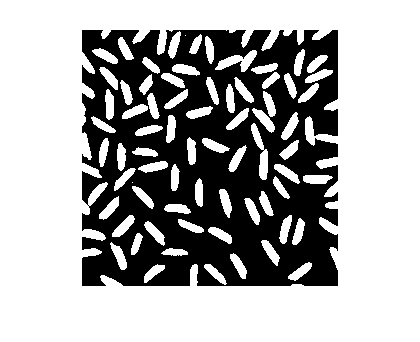Now compute the connected components and the corresponding label matrix.

cc = bwconncomp(bw);
L = labelmatrix(cc);

In the label matrix, each foreground object in the original binary image is assigned a unique positive integer. Here, for instance, is how to display the tenth object.

imshow(L == 10)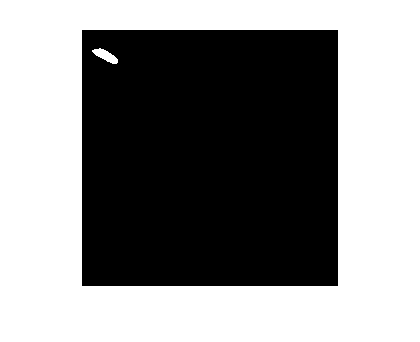Use the function label2rgb to "colorize" the label matrix.

rgb = label2rgb(L);
imshow(rgb)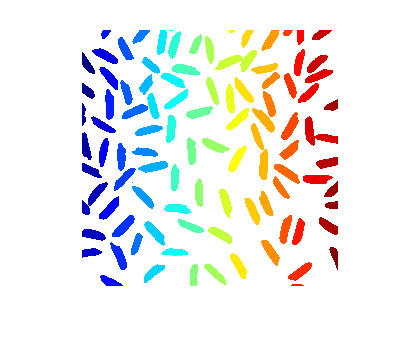That's a nice effect, but because of the way the colors are assigned, object near each other tend to have very similar colors. It might be better sometimes to assign the colors differently. That's what the 'shuffle' argument to label2rgb is for.

Here's the full syntax including 'shuffle':

rgb = label2rgb(L,map,zerocolor,'shuffle');

zerocolor is a three-element vector specifying what color is used for the background pixels.

Let's try it with the jet colormap and a light gray background color.

rgb = label2rgb(L,'jet',[.7 .7 .7],'shuffle');
imshow(rgb)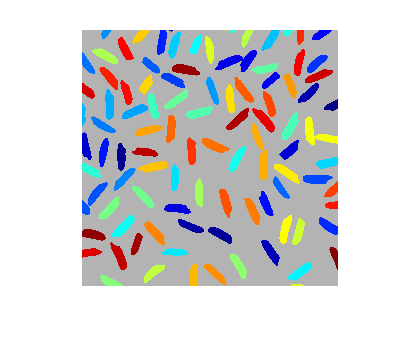Now it's easier to see where two objects might be actually touching and so receive the same label. Let's zoom in closer to see:

xlim([128 185]);
ylim([5 62]);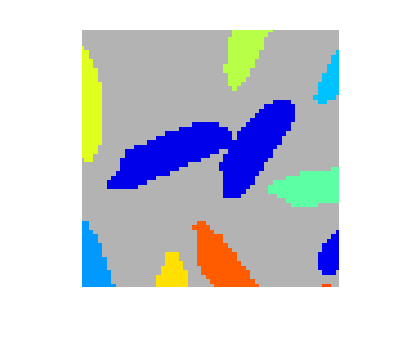I hope you find this useful!

Published with MATLAB® 7.14

|